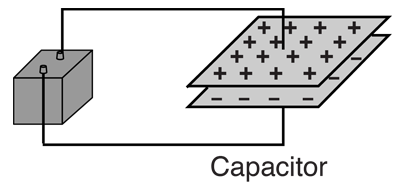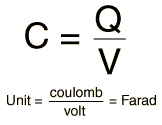# Capacitors

Capacitance is typified by a parallel plate arrangement and is defined in terms of charge storage:A battery will transport charge from one plate to the other until the voltage produced by the charge buildup is equal to the battery voltage.where Q = magnitude of charge stored on each plate. V = voltage applied to the plates.
 Air tank analogy Capacitor combinations Condenser microphone
Index

Capacitance concepts

Inductance concepts

 HyperPhysics***** Electricity and Magnetism R Nave
Go Back

# Capacitor CombinationsIf C1 = μF and C2 = μF
 then Ceq = C1 + C2 + ... = μF

Capacitors in series combine as reciprocals ...Ceq = μF
 Air tank analogy Charge on series capacitors
Index

Capacitance concepts

Inductance concepts

 HyperPhysics***** Electricity and Magnetism R Nave
Go Back

# Charge on Series CapacitorsSince charge cannot be added or taken away from the conductor between series capacitors, the net charge there remains zero. As can be seen from the diagram, that constrains the charge on the two capacitors to be the same in a DC situation. This charge Q is the charge you get by calculating the equivalent capacitance of the series combination and multiplying it by the applied voltage V. You store less charge on series capacitors than you would on either one of them alone with the same voltage!

Does it ever make sense to put capacitors in series? You get less capacitance and less charge storage than with either alone. It is sometimes done in electronics practice because capacitors have maximum working voltages, and with two "600 volt maximum" capacitors in series, you can increase the working voltage to 1200 volts.

 Capacitor combinations
Index

Capacitance concepts

Inductance concepts

 HyperPhysics***** Electricity and Magnetism R Nave
Go Back